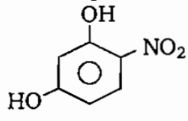The type of molecular forces of attraction present in the following compound is:(a) Intermolecular H-bonding                   (b) Intramolecular H-bonding

(c) van der Waal's force                           (d) All of these

Concept Questions :-

Hydrogen Bonding
High Yielding Test Series + Question Bank - NEET 2020

Difficulty Level:

The species showing $p\pi$-$d\pi$  overlapping is :

(a) $N{{O}_{3}}^{-}$

(b) $P{{O}_{4}}^{3-}$

(c) $C{{O}_{3}}^{2-}$

(d) $N{{O}_{2}}^{-}$

Concept Questions :-

Covalent Bonding
High Yielding Test Series + Question Bank - NEET 2020

Difficulty Level:

Which of the following interaction lies in the range of 8 -  42 kJ/mol?

1. ${\mathrm{H}}_{2}...{\mathrm{H}}_{2}\mathrm{O}$

2. HCl...HCI

3. ${\mathrm{H}}_{2}\mathrm{O}$...HF

4. all

Concept Questions :-

Hydrogen Bonding
High Yielding Test Series + Question Bank - NEET 2020

Difficulty Level:

The number of antibonding electrons pairs in ${{O}_{2}}^{2-}$ on the basis of MO theory are:

(a) 4

(b) 3

(c) 2

(d) 5

Concept Questions :-

M.O.T
High Yielding Test Series + Question Bank - NEET 2020

Difficulty Level:

If ${\mathrm{CdI}}_{2}$ is pink in colour, the ${\mathrm{CdCl}}_{2}$ will be '.............' coloured.

(a) yellow

(b) red

(c) blue

(d) cannot be predicted

Concept Questions :-

Types of Bonding
High Yielding Test Series + Question Bank - NEET 2020

Difficulty Level:

Which are true statements among the following?

(1) $P{H}_{5}$ and $BiC{l}_{5}$ does not exist

(2) $p\pi$-$d\pi$ bonds are present in $S{O}_{2}$

(3) Electrons travel with speed of light

(4) $Se{F}_{4}$ and $C{H}_{4}$ has same changes

(5) ${{I}_{3}}^{+}$ has bent geometry

(a) 1,3

(b) 1,2,5

(c) 1,3,5

(d) 1,2,4

Concept Questions :-

Hybridisation
High Yielding Test Series + Question Bank - NEET 2020

Difficulty Level:

In which molecule all atoms are coplanar?

(a)$C{H}_{4}$

(b) $B{F}_{3}$

(c) $P{F}_{3}$

(d) $N{H}_{3}$

Concept Questions :-

Hybridisation
High Yielding Test Series + Question Bank - NEET 2020

Difficulty Level:

Which species does not exist?

(a) ${\left(SnC{l}_{6}\right)}^{2-}$

(b) ${\left(GeC{l}_{6}\right)}^{2-}$

(c) ${\left(CC{l}_{6}\right)}^{2-}$

(d) ${\left(SiC{l}_{6}\right)}^{2-}$

Concept Questions :-

Hybridisation
High Yielding Test Series + Question Bank - NEET 2020

Difficulty Level:

Which is paramagentic?

(a) $C{l}_{2}{O}_{6}$

(b) $C{l}_{2}{O}_{7}$

(c) $C{l}_{2}O$

(d) $Cl{O}_{2}$

Concept Questions :-

Covalent Bonding
High Yielding Test Series + Question Bank - NEET 2020

Difficulty Level:

Greater the dipole moment:

(a) greater is the ionic nature

(b) lesser the polarity

(c) smaller is the ionic nature

(d) none of these

Concept Questions :-

Polarity